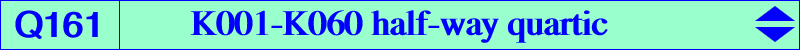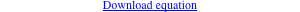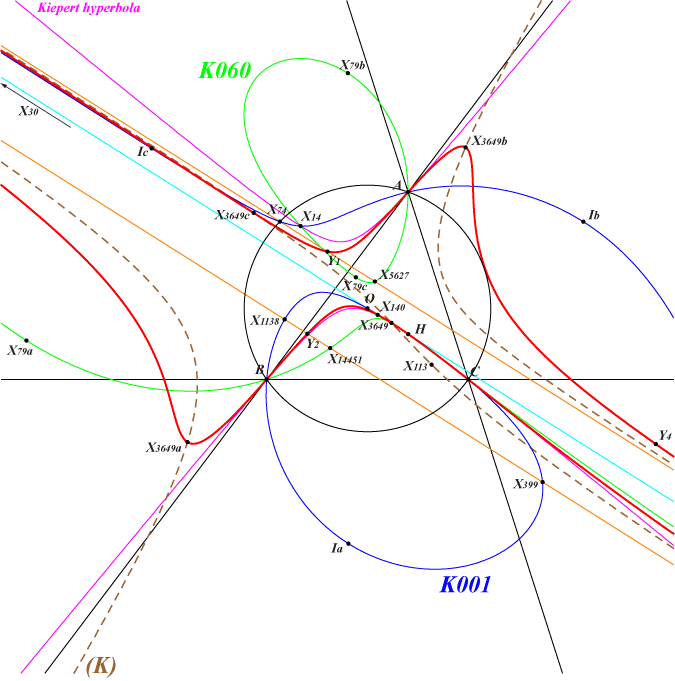too complicated to be written here. Click on the link to download a text file.X(4), X(30), X(140), X(298), X(299), X(395), X(396), X(523), X(3649), X(34325), X(34326), X(40630), X(40631), X(40662), X(40663), X(40664), X(40665), X(40666), X(40667), X(40668), X(40669) Y1 = midpoint of X(74), X(5627) = X(40630) Y2 = midpoint of X(1138), X(14451) = X(40662) Y3 = midpoint of X(80), X(484) = X(40663) Y4 = midpoint of X(1141), X(1157) = X(40631) extraversions of X(3649) and X(40663)(This page was written from an idea by Francisco Javier García Capitán and with his active cooperation) Let P be a point with pedal triangle PaPbPc and denote by Oa, Ob, Oc the midpoints of AP, BP, CP respectively. Note that Oa is the circumcenter of triangle APbPc. If Qa, Qb, Qc are the reflections of P in the sidelines of ABC then the lines OaPa, ObPb, OcPc concur (at Q) if and only if AQa, BQb, CQc concur (at R) which shows that P must lie on the Neuberg cubic K001 and R on K060. Q is the midpoint of PR and lies on the quartic Q161. The points P, R, Q and the isogonal conjugate P* of P lie on a same parallel to the Euler line. These points P, P* (on K001) are labelled P1, P2 on the figure, with corresponding points R1, R2 on K060 and Q1, Q2 on Q161. Q161 has two pairs of parallel asymptotes : • two asymptotes are real and parallel to the Euler line at X(74) and X(399). These are actually the real asymptotes of K001 and K060 respectively. Each asymptote meets Q161 at X(30) counted three times and a fourth finite point. These are Y1, Y2 on the figure. • two asymptotes are imaginary and perpendicular to the Euler line. The parallels to the Euler line at the in/excenters of ABC are tangent to the three curves K001, K060, Q161 at X(1), X(79), X(3649) and extraversions respectively. Recall that X(3649) is the midpoint of X(1), X(79) and the same applies to extraversions. The tangents to Q161 at A, B, C, H are those to the Kiepert hyperbola which thus meets Q161 at four points, each counted twice. The tangent at H passes through X(6) and meets Q161 again at X(40665), X(40666).(K) is the third polar curve of X(30) in Q161 i.e. the locus of points whose polar line is parallel to the Euler line. It is a cubic having the same asymptotes parallel to the Euler line as K001, K060, Q161 and a third asymptote which is the line passing through X(125), X(523). Any line parallel to the Euler line meets Q161 at X(30) at least twice and two other points whose midpoint is on (K). (K) passes through X(30), X(113), X(523), X(3850), X(3649) and its extraversions. *** The following table shows a selection of points P, R, Q on K001, K060, Q161 respectively. Recall that Q is the midpoint of P, R.P X(1) X(3) X(4) X(13) X(14) X(15) X(16) X(30) X(74) X(399) X(484) X(616) R X(79) X(5) X(4) X(11581) X(11582) X(13) X(14) X(265) X(5627) X(30) X(80) X(621) Q X(3649) X(140) X(4) X(34325) X(34326) X(396) X(395) X(30) X(40630) X(30) X(40663) X(298)P X(617) X(1138) X(1157) X(1263) X(1276) X(1277) X(1337) X(1338) X(2132) X(2133) X(3065) X(3440) R X(622) X(14451) X(1141) X(34302) ? ? ? ? X(34298) ? X(14452) X(34296) Q X(299) X(40662) X(40631) X() X() X() X() X() X() X() X() X()P X(3441) X(3464) X(3465) X(3466) X(3479) X(3480) X(3481) X(3482) X(3483) X(3484) X(5623) X(5624) R X(34295) X(34301) X(34300) X(34299) X(14373) X(14372) X(33664) ? X(34305) X(34304) X() X() Q X() X(40669) X() X() X() X() X() X() X() X() X() X()P X(5667) X(5668) X(5669) X(5671) X(5674) X(5675) X(5684) X(5685) X(7165) X(8172) X(8173) X(8431) R X(6761) X(38943) X(38944) X(1117) X(39132) X(39133) X(11584) X(19658) X(34303) X(11600) X(11601) X(34297) Q X(40664) X(40665) X(40666) X() X() X() X() X() X() X(40667) X(40668) X()P X(8437) X(8438) X(8444) X(8454) X(16882) X(16883) X() X() X() X() X() X() R X(39134) X(39135) ? ? X(17405) X(17406) X() X() X() X() X() X() Q X() X() X() X() X() X() X() X() X() X() X() X()